pyclustering.cluster.xmeans.splitting_type Class Reference

Enumeration of splitting types that can be used as splitting creation of cluster in X-Means algorithm. More...Inheritance diagram for pyclustering.cluster.xmeans.splitting_type:Collaboration diagram for pyclustering.cluster.xmeans.splitting_type:

## Static Public Attributes

int BAYESIAN_INFORMATION_CRITERION = 0
Bayesian information criterion (BIC) to approximate the correct number of clusters. More...

int MINIMUM_NOISELESS_DESCRIPTION_LENGTH = 1
Minimum noiseless description length (MNDL) to approximate the correct number of clusters. More...

## Detailed Description

Enumeration of splitting types that can be used as splitting creation of cluster in X-Means algorithm.

Definition at line 46 of file xmeans.py.

## ◆ BAYESIAN_INFORMATION_CRITERION

 int pyclustering.cluster.xmeans.splitting_type.BAYESIAN_INFORMATION_CRITERION = 0
static

Bayesian information criterion (BIC) to approximate the correct number of clusters.

Kass's formula is used to calculate BIC: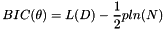The number of free parameters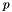is simply the sum of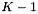class probabilities,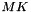centroid coordinates, and one variance estimate: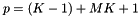The log-likelihood of the data: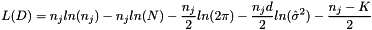The maximum likelihood estimate (MLE) for the variance: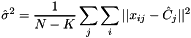Definition at line 64 of file xmeans.py.

## ◆ MINIMUM_NOISELESS_DESCRIPTION_LENGTH

 int pyclustering.cluster.xmeans.splitting_type.MINIMUM_NOISELESS_DESCRIPTION_LENGTH = 1
static

Minimum noiseless description length (MNDL) to approximate the correct number of clusters.

Beheshti's formula is used to calculate upper bound: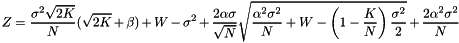where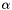and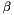represent the parameters for validation probability and confidence probability.

To improve clustering results some contradiction is introduced: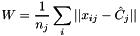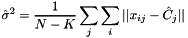Definition at line 75 of file xmeans.py.

The documentation for this class was generated from the following file: